Home Practice
For learners and parents For teachers and schools
Textbooks
Full catalogue
Pricing SupportLog in

We think you are located in United States. Is this correct?

# 10.2 Series and parallel resistor networks (Revision)

## 10.2 Series and parallel resistor networks (Revision) (ESCPT)

In Grade 10 and Grade 11 you learnt about electric circuits and we introduced three quantities which are fundamental to dealing with electric circuits. These quantities are closely related and are current, voltage (potential difference) and resistance. To recap:

1. Electrical current, $$I$$, is defined as the rate of flow of charge through a circuit.

2. Potential difference or voltage, $$V$$, is related to the energy gained or lost per unit charge moving between two points in a circuit. Charge moving through a battery gains energy which is then lost moving through the circuit.

3. Resistance, $$R$$, is an internal property of a circuit element that opposes the flow of charge. Work must be done for a charge to move through a resistor.

These quantities can be related, in circuit elements whose resistance remains constant, by Ohm's law.

Ohm's Law

For a resistor at constant temperature the ratio $$\frac{V}{I}$$ is constant. This ratio we call resistance.

This is equivalent to saying that the amount of electric current through a metal conductor, at a constant temperature, in a circuit is proportional to the voltage across the conductor and can be described by

$I = \frac{V}{R}$

In other words, at constant temperature, the resistance of the conductor is constant, independent of the voltage applied across it or current passed through it.

We have focused on the properties of a single component. Now we need to look at a collection of components in a circuit.

You will often hear people switch between using the terms voltage and potential difference to describe the same quantity. This is correct but very important to note.

Circuits don't consist of a single element and we've learnt about how voltage, current and resistance are affected in circuits with multiple resistors. There are two basic layouts we consider for a network of resistors, series and parallel. Resistors in series and resistors in parallel have different features when talking about current, voltage and equivalent resistance.

## Worked example 1: Ohm's Law [NSC 2011 Paper 1]

Learners conduct an investigation to verify Ohm's law. They measure the current through a conducting wire for different potential differences across its ends. The results obtained are shown in the graph below.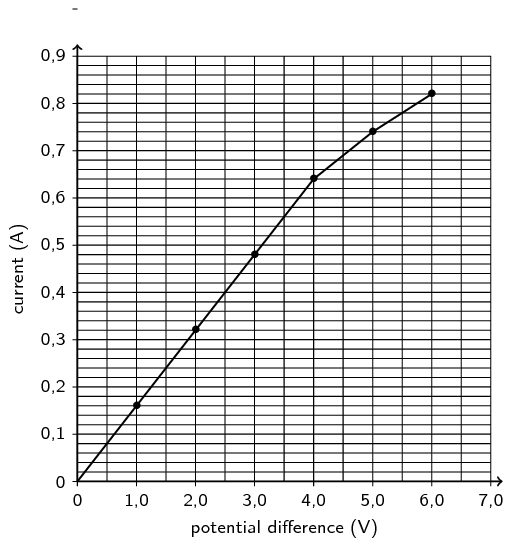1. Which ONE of the measured quantities is the dependent variable?

(1 mark)

2. The graph deviates from Ohm's law at some point.

1. Write down the coordinates of the plotted point on the graph beyond which Ohm's law is not obeyed.

(2 marks)

2. Give a possible reason for the deviation from Ohm's law as shown in the graph. Assume that all measurements are correct.

(2 marks)

3. Calculate the gradient of the graph for the section where Ohm's law is obeyed.

Use this to calculate the resistance of the conducting wire.

(4 marks)

Question 1

Current OR I

(1 mark)

Question 2

The graph deviates from Ohm's law at some point.

1. ($$\text{4,0}$$ ; $$\text{0,64}$$)

(2 marks)

2. Temperature was not kept constant.

(2 marks)

Question 3

\begin{align*} \text{Gradient:} \quad m & = \frac{\Delta y}{\Delta x} \\ & = \frac{\text{0,64}-\text{0}}{\text{4}-\text{0}} \\ & = \text{0,16} \end{align*}\begin{align*} R & = \frac{1}{\text{0,16}} \\ & = \text{6,25}\ \Omega \end{align*}

(4 marks)

[TOTAL: 9 marks]

temp text

A circuit may consist of a combination of parallel and series networks that can in turn be in parallel or series. We can treat parts of the total circuit independently by applying Ohm's Law to each of the components.

Resistors connected in series

Resistors are in series if they are consecutive elements in the sequence of the circuit and there are no branches between them. For $$n$$ resistors in series the equivalent resistance is:

$R_{s} = R_{1} + R_{2} + R_{3} + \ldots + R_{n}$

For $$n$$ resistors in series the potential difference is split across the resistors:

$V_{\text{Total}} = V_{1} + V_{2} + V_{3} + \ldots + V_{n}$

The current is constant through the resistors.

$I_{\text{Total}} = I_{1} = I_{2} = I_{3} = \ldots = I_{n}$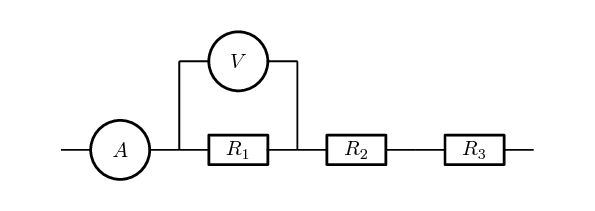It makes sense to remind ourselves of why these are consistent with other topics we have covered previously:

• Conservation of charge: we have learnt that charges are not created or destroyed. This is consistent with the current being the same throughout a resistor network that is in series. Charges aren't being added or lost or bunching up and, therefore, the rate at which charge moves past each point should be the same.
• Conservation of energy: we have learnt that energy isn't created or destroyed but transferred through work. The voltage across a resistor is the energy per unit charge (work) required to move through the resistor. The total work done to move through a network of resistors in series should be the sum of the work done to move through each individual resistor.

## Worked example 2: Ohm's Law, all components in series

Two ohmic resistors ($$R_{1}$$ and $$R_{2}$$) are connected in series with a cell with negligible internal resistance. Find the resistance of $$R_{2}$$, given that the current flowing through $$R_{1}$$ and $$R_{2}$$ is $$\text{0,25}$$ $$\text{A}$$ and that the potential difference across the cell is $$\text{1,50}$$ $$\text{V}$$. $$R_{1}$$ =$$\text{1,00}$$ $$\text{Ω}$$.

### Draw the circuit and fill in all known values.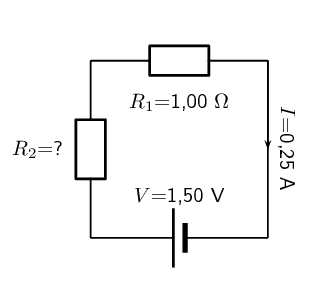### Determine how to approach the problem.

We can use Ohm's Law to find the total resistance R in the circuit, and then calculate the unknown resistance using:

$R = R_{1} + R_{2}$

because $$R_{1}$$ and $$R_{2}$$ are connected in series.

### Find the total resistance

\begin{align*} R & = \frac{V}{I} \\ & = \frac{\text{1,5}}{\text{0,25}} \\ & = \text{6}\text{ Ω} \end{align*}

### Find the unknown resistance

We know that:

$R = \text{6,00}\text{ Ω}$

and that

$R_{1} = \text{1,00}\text{ Ω}$

Since

$R = R_{1} + R_{2}$ $R_{2} = R - R_{1}$

Therefore,

$R_{1} = \text{5,00}\text{ Ω}$

## Worked example 3: Ohm's Law, series circuit

In the case of the circuit shown, calculate:

1. the potential difference of $$V_1$$, $$V_2$$ and $$V_3$$ across the resistors $$R_1$$, $$R_2$$, and $$R_3$$

2. the resistance of $$R_3$$.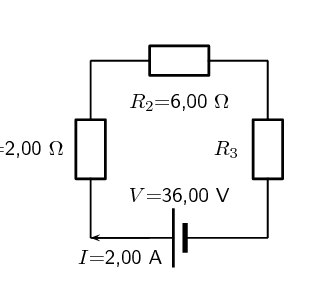### Determine how to approach the problem

We are given the potential difference across the cell and the current in the circuit, as well as the resistances of two of the three resistors. We can use Ohm's Law to calculate the potential difference across the known resistors. Since the resistors are in a series circuit the potential difference is $$V = V_1 + V_2 + V_3$$ and we can calculate $$V_3$$. Now we can use this information to find the potential difference across the unknown resistor $$R_3$$.

### Calculate potential difference across $$R_1$$

Using Ohm's Law: \begin{align*} R_1 &= \frac{V_1}{I} \\ I \cdot R_1 &= I \cdot \frac{V_1}{I} \\ V_1 &= {I}\cdot{R_1}\\ &= \text{2} \cdot \text{2} \\ V_1 &= \text{4,00}\text{ V} \end{align*}

### Calculate potential difference across $$R_2$$

Use Ohm's Law: \begin{align*} R_2 &= \frac{V_2}{I} \\ I \cdot R_2 &= I \cdot \frac{V_2}{I} \\ V_2 &= {I}\cdot{R_2}\\ &= \text{2} \cdot \text{6} \\ V_2 &= \text{12,00}\text{ V} \end{align*}

### Calculate potential difference across $$R_3$$

Since the potential difference across all the resistors combined must be the same as the potential difference across the cell in a series circuit, we can find $$V_3$$ using: \begin{align*} V &= V_1 + V_2 + V_3\\ V_3 &= V - V_1 - V_2 \\ &= \text{36} - \text{4} - \text{12} \\ V_3&= \text{20,00}\text{ V} \end{align*}

### Find the resistance of $$R_3$$

We know the potential difference across $$R_3$$ and the current through it, so we can use Ohm's Law to calculate the value for the resistance: \begin{align*} R_3 &= \frac{V_3}{I}\\ &= \frac{ \text{20}}{ \text{2}} \\ R_3&= \text{10,00}~\Omega \end{align*}

• $$V_1 = \text{4,00}\text{ V}$$
• $$V_2 = \text{12,00}\text{ V}$$
• $$V_3 = \text{20,00}\text{ V}$$
• $$R_3 = \text{10,00}~\Omega$$

Equivalent resistance in a parallel network

A parallel configuration is when the current splits into a number of branches which contain components (resistors in our case). A branch may contain multiple resistors in series and still be part of the parallel configuration. For $$n$$ branches of resistors in parallel, the equivalent resistance can be calculated from the total resistance of each branch and is:

$\frac{1}{R_{p}} = \frac{1}{R_{B1}} + \frac{1}{R_{B2}} + \frac{1}{R_{B3}} + \ldots + \frac{1}{R_{Bn}}$

For $$n$$ branches of resistors in parallel the potential difference is the same across each of the branches:

$V_{\text{Total}} = V_{B1} = V_{B2} = V_{B3} = \ldots = V_{Bn}$

The current is split through the branches.

$I_{\text{Total}} = I_{1} + I_{2} + I_{3} + \ldots + I_{n}$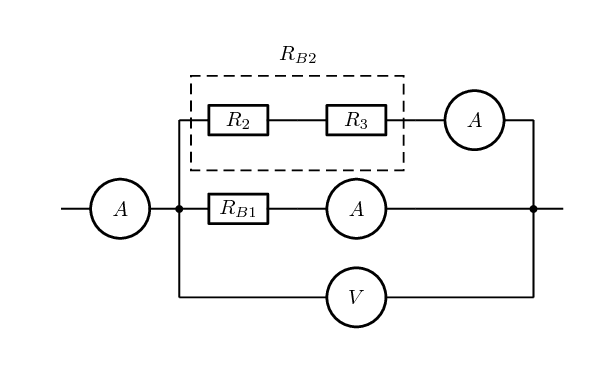temp text

Let's take a moment to see if our conservation laws still make sense:

• Conservation of charge: we have learnt that charges are not created or destroyed. This is consistent with the current splitting between the branches. Charges aren't being added or lost or bunching up and, therefore, the total number of charges going through the branches must be the same as the number entering the point where the circuit branches.
• Conservation of energy: we have learnt that energy isn't created or destroyed but transferred through work. Energy per unit charge doesn't change unless work is done therefore it makes sense that the energy per unit charge in each branch should be the same.

## Worked example 4: Ohm's Law, resistors connected in parallel

Calculate the current ($$I$$) in this circuit if the resistors are both ohmic in nature.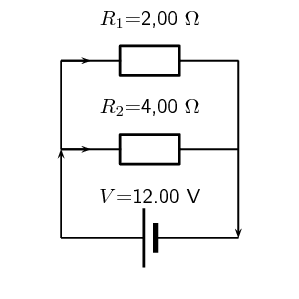### Determine what is required

We are required to calculate the total current flowing in the circuit.

### Determine how to approach the problem

Since the resistors are ohmic in nature, we can use Ohm's Law. However, there are two resistors in the circuit and we need to find the total resistance.

### Find the equivalent resistance in the circuit

Since the resistors are connected in parallel, the total (equivalent) resistance R is:

$\frac{1}{R} = \frac{1}{R_{1}} + \frac{1}{R_{2}}.$ \begin{align*} \frac{1}{R} &= \frac{\text{1}}{R_1} + \frac{\text{1}}{R_2} \\ &= \frac{\text{1}}{\text{2}} + \frac{\text{1}}{\text{4}} \\ &= \frac{\text{2}+\text{1}}{\text{4}} \\ &= \frac{\text{3}}{\text{4}} \\ \text{Therefore, } R &= \frac{\text{4}}{\text{3}} = \text{1,33}~\Omega \end{align*}

### Apply Ohm's Law

\begin{align*} R&= \frac{V}{I} \\ R \cdot \frac{I}{R} &= \frac{V}{I} \cdot \frac{I}{R} \\ I &= \frac{V}{R}\\ I &= V \cdot \frac{\text{1}}{R}\\ &= (\text{12}) \left(\frac{\text{3}}{\text{4}}\right) \\ &= \text{9,00}\text{ A} \end{align*}

The total current flowing in the circuit is $$\text{9,00}$$ $$\text{A}$$.

## Worked example 5: Ohm's Law, parallel network of resistors

An $$\text{18,00}$$ $$\text{V}$$ cell is connected to two parallel resistors of $$\text{2,00}$$ $$\Omega$$ and $$\text{6,00}$$ $$\Omega$$ respectively. Calculate the current through each of the ammeters when the switch is closed and when it is open.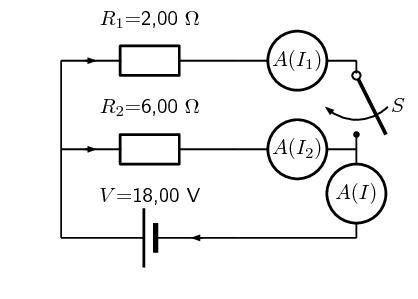### Determine how to approach the problem

We need to determine the current through the cell and each of the parallel resistors. We have been given the potential difference across the cell and the resistances of the resistors, so we can use Ohm's Law to calculate the current.

There are two alternative approaches we could adopt:

• we could use the fact that the potential difference across each of the resistors is the same as the potential difference across the battery because they are in a parallel configuration and then use Ohm's Law; or
• we could determine the equivalent resistance of the circuit and the total current and then use that to determine the current through each of the resistors.

Both methods will result in the correct answer if you don't make any calculation errors but one is shorter.

### Now determine the current through one of the parallel resistors

We know that for a configuration with just two resistors in parallel and a cell as in this case, the potential difference across the cell is the same as the potential difference across each of the resistors in parallel. For this circuit: \begin{align*} V &= V_1 = V_2 = \text{18,00}\text{ V} \end{align*} Let's start with calculating the current through $$R_1$$ using Ohm's Law: \begin{align*} R_1 &= \frac{V_1}{I_1} \\ I_1 &= \frac{V_1}{R_1} \\ &= \frac{\text{18,00}}{\text{2,00}} \\ I_1 &= \text{9,00}\text{ A} \end{align*}

### Calculate the current through the other parallel resistor

We can use Ohm's Law again to find the current in $$R_2$$: \begin{align*} R_2 &= \frac{V_2}{I_2} \\ I_2 &= \frac{V_2}{R_2} \\ &= \frac{\text{18,00}}{\text{6,00}} \\ I_2 &= \text{3,00}\text{ A} \end{align*}

### Calculate the total current

The current through each of the parallel resistors must add up to the total current through the cell: \begin{align*} I &= I_1 + I_2 \\ &= \text{9,00} + \text{3,00} \\ I_2 &= \text{12,00}\text{ A} \end{align*}

### When the switch is open

The branch through $$R_1$$ is not complete so no current can flow through it. This means we can ignore it completely and consider a simple circuit with a battery and a single resistor, $$R_2$$, in it.

We can use Ohm's Law again to find the current in $$R_2$$: \begin{align*} R_2 &= \frac{V_2}{I_2} \\ I_2 &= \frac{V_2}{R_2} \\ &= \frac{\text{18,00}}{\text{6,00}} \\ I_2 &= \text{3,00}\text{ A} \end{align*}

When the switch is closed:

• The current through the cell is $$\text{12,00}$$ $$\text{A}$$.
• The current through the $$\text{2,00}$$ $$\Omega$$ resistor is $$\text{9,00}$$ $$\text{A}$$.
• The current through the $$\text{6,00}$$ $$\Omega$$ resistor is $$\text{3,00}$$ $$\text{A}$$.

When the switch is open:

• The current through the $$\text{6,00}$$ $$\Omega$$ resistor is $$\text{3,00}$$ $$\text{A}$$.
temp text

## Series and parallel networks

### Aim

To investigate the changes in current and voltage when branches of circuits are open or closed..

### Apparatus

You will need the following items for this investigation:

• batterys / cells

• a set of resistors and/or light bulbs

• ammeters

• voltmeters

### Method

For this investigation, configure a circuit with resistors in both series and in parallel. For example, try:

• including parallel branches with different numbers of light bulbs in each branch
• changing the numbers of light bulbs or resistors in each branch
• try adding a resistor in series with the parallel network
In each branch include an ammeter and a switch. Make notes about what happens when you remove a branch by opening the switch in the branch. What happens to the current in the other branches. Try to predict what will happen before opening or closing a switch and before adding or removing any light bulbs or resistors.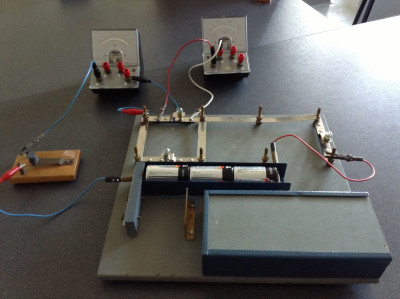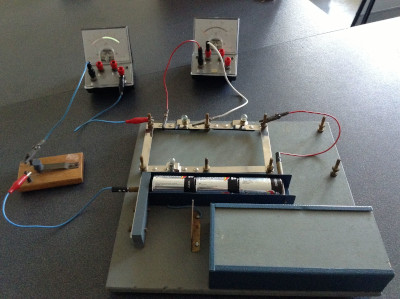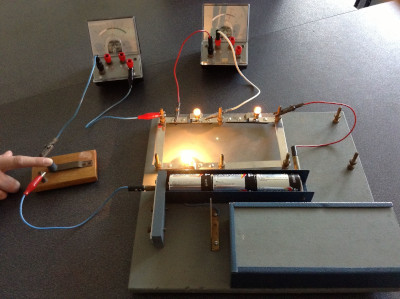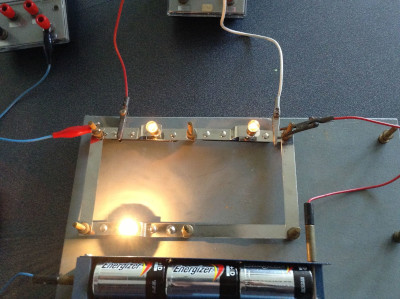### Discussion

Be sure to note and discuss:

• whether adding a new branch increases or decreases the total current in the circuit,
• whether adding a new branch increases or decreases the current in the original branches,
• whether adding a resistor in series with a parallel network increases or decreases the current, and
• compare what happens when you add a resistor in series with adding another branch to the parallel network.

## Series and parallel networks

Textbook Exercise 10.1

The diagram shows an electric circuit consisting of a battery and four resistors.

The potential difference (voltage) over the battery is $$V_A = \text{2,8}\text{ V}$$

The resistors are rated as follows:

• $$R_1 = \text{7,2}\text{ Ω}$$
• $$R_2 = \text{4,3}\text{ Ω}$$
• $$R_3 = \text{7,5}\text{ Ω}$$
• $$R_4 = \text{4,1}\text{ Ω}$$

Assume that positive charge is flowing in the circuit (conventional current).

Using the concepts of Ohm's law, and electric circuits, determine the following:

What type of circuit is shown in the diagram?

We need to determine whether the circuit is a series, parallel, or combination type of circuit. We do this by looking at how current flows through a circuit.

Recall that current is the movement of electric charge from a higher potential to a lower potential.

We assume that the flowing charge is positive (conventional current). This means that the charges start at the positive terminal of our power source (battery). Here the charges have lots of electrical potential energy.

The charges then move towards the negative terminal through the path that is created by the components and wires of the circuit. During this process, the electrical potential energy is converted into thermal energy by the resistors. Therefore, the charges have less electrical potential energy when they reach the negative terminal of the power source (battery).

The flow of current is indicated by the arrows in the diagram below:

From the diagram it is clear that there is only one path for the current to flow, since the circuit does not split into two or more paths. This means that the current flows through all the components, one after the other (in series). We will label this current $$I_A$$.

The circuit shown in the diagram is a series circuit.

$$0$$

What is the total equivalent resistance $$R_{eq}$$ of the circuit?

• use the values for any physical constants you might need, as listed here

We know that the circuit in the diagram is a series type circuit.

Recall that in a series circuit the equivalent resistance can be calculated by summing the resistance values of the individual resistors:

$R_{eq,S} = R_1 + R_2 + R_3 + \ldots ~~~~~\text{(1)}$

We can rewrite $$\text{(1)}$$ for the circuit in question as:

$R_{eq} = R_1 + R_2 + R_3 + R_4 ~~~~~\text{(2)}$

We can represent this by drawing an equivalent circuit:

We are given the following information:

• the resistance of $$R_1 = \text{7,2}\text{ Ω}$$
• the resistance of $$R_2 = \text{4,3}\text{ Ω}$$
• the resistance of $$R_3 = \text{7,5}\text{ Ω}$$
• the resistance of $$R_4 = \text{4,1}\text{ Ω}$$

Substituting the above values in $$\text{(2)}$$, we get:

\begin{align*} R_{eq} & = R_1 + R_2 + R_3 + R_4\\ & = \text{7,2} + \text{4,3} + \text{7,5} + \text{4,1}\\ & = \text{23,1}\text{ Ω} \end{align*}

Therefore, the total equivalent resistance in the circuit, or $$R_{eq} = \text{23,1}\text{ Ω}$$

$$\text{23,1}$$

What is the potential difference (voltage) across $$R_1$$, or $$V_1$$ ?

• use the values for any physical constants you might need, as listed here

Potential difference, or voltage is a way to describe the difference in electrical potential energy across a component in a circuit. Remember that a resistor converts electrical potential energy into thermal energy, so the electrical potential is higher on the "in" side compared to the "out" side of a resistor.

Ohm's law describes the relationship between the total current $$~I~$$ through an ohmic conductor, its resistance $$R$$, and the potential difference $$V~$$ across it:

$I = \frac{V}{R}~~~~~\text{(3)}$

For the equivalent circuit, we can rewrite $$\text{(3)}$$ as:

$I_A = \frac{V_A}{R_{eq}}~~~~~\text{(4)}$

We are asked to calculate the potential difference $$V_{1}$$ over $$R_{1}$$, as shown in the circuit diagram above, so we use Ohm's law and rewrite $$\text{(3)}$$ to get:

$V_1 = I_1R_1~~~~~\text{(5)}$

Since the circuit in question is a series circuit, we know that the current $$I_{1}$$ flowing through $$R_{1}$$, is the same as the current $$I_{A}$$. This is represented as:

$I_1 = I_A~~~~~\text{(6)}$

Substituting $$\text{(6)}$$ into $$\text{(5)}$$ we get:

$V_1 = I_AR_1~~~~~\text{(7)}$

Substituting $$\text{(4)}$$ into $$\text{(7)}$$ we get:

$V_{1} = I_AR_1 = \left(\frac{V_A}{R_{eq}}\right)R_1 = \frac{V_AR_1}{R_{eq}}~~~~~\text{(8)}$

Recall from Question 2:

$R_{eq} = R_1 + R_2 + R_3 + R_4 ~~~~~\text{(2)}$

Substituting $$\text{(2)}$$ into $$\text{(8)}$$ we get:

$V_{1} = \frac{V_AR_1}{R_1 + R_2 + R_3 + R_4}~~~~~\text{(9)}$

We are given the following information:

• the resistance of $$R_1 = \text{7,2}\text{ Ω}$$
• the resistance of $$R_2 = \text{4,3}\text{ Ω}$$
• the resistance of $$R_3 = \text{7,5}\text{ Ω}$$
• the resistance of $$R_4 = \text{4,1}\text{ Ω}$$
• the potential difference (voltage) over the battery $$V_A = \text{2,8}\text{ V}$$

Substituting the above values in $$\text{(9)}$$, we get:

\begin{align*} V_{1} & = \frac{V_AR_1}{R_1 + R_2 + R_3 + R_4}\\ & = \frac {(\text{2,8})(\text{7,2})} {\text{7,2} + \text{4,3} + \text{7,5} + \text{4,1}}\\ & = 0,87273 \ldots~~~~~\text{(calculated)}\\ & \approx \text{0,873}\text{ V}~~~~~\text{(rounded)} \end{align*}

Therefore, the potential difference (voltage) across $$R_1$$, or $$V_1 \approx \text{0,873}\text{ V}$$.

$$\text{0,873}$$

What is the potential difference (voltage) across $$R_2$$, $$R_3$$, and $$R_4$$, or $$V_2$$, $$V_3$$, and $$V_4$$?

• use the values for any physical constants you might need, as listed here

We are asked to calculate the three remaining potential differences, $$V_2$$, $$V_3$$, and $$V_4$$. This is shown in the diagram below:

We will use the same method to obtain three expression for $$V_2$$, $$V_3$$, and $$V_4$$ as was used in Question 3 for $$V_1$$. We get:

$V_{2} = \frac{V_AR_2}{R_1 + R_2 + R_3 + R_4}~~~~~\text{(10)}$

$V_{3} = \frac{V_AR_3}{R_1 + R_2 + R_3 + R_4}~~~~~\text{(11)}$

$V_{4} = \frac{V_AR_4}{R_1 + R_2 + R_3 + R_4}~~~~~\text{(12)}$

We are given the following information:

• the resistance of $$R_1 = \text{7,2}\text{ Ω}$$
• the resistance of $$R_2 = \text{4,3}\text{ Ω}$$
• the resistance of $$R_3 = \text{7,5}\text{ Ω}$$
• the resistance of $$R_4 = \text{4,1}\text{ Ω}$$
• the potential difference (voltage) over the battery $$V_A = \text{2,8}\text{ V}$$

Substituting the above values in $$\text{(10)}$$, $$\text{(11)}$$, and $$\text{(12)}$$ we get:

\begin{align*} V_{2} & = \frac{V_AR_2}{R_1 + R_2 + R_3 + R_4}\\ & = \frac {(\text{2,8})(\text{4,3})} {\text{7,2} + \text{4,3} + \text{7,5} + \text{4,1}}\\ & = 0,52121 \ldots~~~~~\text{(calculated)}\\ & \approx \text{0,521}\text{ V}~~~~~\text{(rounded)} \end{align*}

\begin{align*} V_{3} & = \frac{V_AR_3}{R_1 + R_2 + R_3 + R_4}\\ & = \frac {(\text{2,8})(\text{7,5})} {\text{7,2} + \text{4,3} + \text{7,5} + \text{4,1}}\\ & = 0,90909 \ldots~~~~~\text{(calculated)}\\ & \approx \text{0,909}\text{ V}~~~~~\text{(rounded)} \end{align*}

\begin{align*} V_{4} & = \frac{V_AR_4}{R_1 + R_2 + R_3 + R_4}\\ & = \frac {(\text{2,8})(\text{4,1})} {\text{7,2} + \text{4,3} + \text{7,5} + \text{4,1}}\\ & = 0,49697 \ldots~~~~~\text{(calculated)}\\ & \approx \text{0,497}\text{ V}~~~~~\text{(rounded)} \end{align*}

Therefore, the potential difference (voltage) across $$R_2$$, or $$V_2 \approx \text{0,521}\text{ V}$$.

The potential difference (voltage) across $$R_3$$, or $$V_3 \approx \text{0,909}\text{ V}$$.

The potential difference (voltage) across $$R_4$$, or $$V_4 \approx \text{0,497}\text{ V}$$.

$$\text{0,521}$$ $$\text{0,909}$$ $$\text{0,497}$$

For the following circuit, calculate: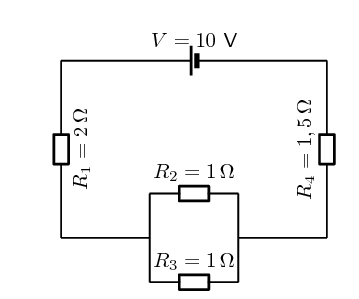the current through the cell

To find the current we first need to find the equivalent resistance. We start by calculating the equivalent resistance of the parallel combination:

\begin{align*} \frac{1}{R_{p}} & = \frac{1}{R_{2}} + \frac{1}{R_{3}} \\ & = \frac{1}{1} + \frac{1}{1} \\ & = 2\\ R_{p} & = \text{0,5}\text{ Ω} \end{align*}

Now we have a circuit with two resistors in series so we can calculate the equivalent resistance:

\begin{align*} R_{s} & = R_{1} + R_{4} + R_{p} \\ & = \text{2} + \text{1,5} + \text{0,5} \\ & = \text{4}\text{ Ω} \end{align*}

So the current through the cell is:

\begin{align*} I & = \frac{V}{R} \\ & = \frac{\text{10}}{\text{4}} \\ & = \text{2,5}\text{ A} \end{align*}

the potential difference across $$R_4$$

The current through all the resistors is $$\text{2,5}$$ $$\text{A}$$. (The current is the same through series combinations of resistors and we can consider the entire parallel set of resistors as one series resistor.)

Using this we can find the potential difference through $$R_{4}$$:

\begin{align*} V & = I \cdot R \\ & = (\text{2,5})(\text{1,5}) \\ & = \text{3,75}\text{ V} \end{align*}

the current through $$R_2$$

The current through all the resistors is $$\text{2,5}$$ $$\text{A}$$. (The current is the same through series combinations of resistors and we can consider the entire parallel set of resistors as one series resistor.)

Using this we can find the current through $$R_{2}$$.

We first need to find the potential difference across the parallel combination:

\begin{align*} V & = I \cdot R \\ & = (\text{2,5})(\text{0,5}) \\ & = \text{1,25}\text{ V} \end{align*}

Now we can find the current through $$R_{2}$$ using the fact that the potential difference is the same across each resistor in the parallel combination:

\begin{align*} I & = \frac{V}{R} \\ & = \frac{\text{1,25}}{\text{1}} \\ & = \text{1,25}\text{ A} \end{align*}

Calculate the equivalent resistance of:

1. three $$\text{2}$$ $$\text{Ω}$$ resistors in series;

2. two $$\text{4}$$ $$\text{Ω}$$ resistors in parallel;

3. a $$\text{4}$$ $$\text{Ω}$$ resistor in series with a $$\text{8}$$ $$\text{Ω}$$ resistor;

4. a $$\text{6}$$ $$\text{Ω}$$ resistor in series with two resistors ($$\text{4}$$ $$\text{Ω}$$ and $$\text{2}$$ $$\text{Ω}$$) in parallel.

1. three $$\text{2}$$ $$\text{Ω}$$ resistors in series:

\begin{align*} R_T&= R_1 + R_2 + R_3 \\ &= (2) + (2) + (2) \\ &= \text{6}\text{ Ω} \end{align*}
2. two $$\text{4}$$ $$\text{Ω}$$ resistors in parallel:

\begin{align*} \frac{1}{R_T}&= \frac{1}{R_1} + \frac{1}{R_2} \\ \frac{1}{R_T}&= \frac{1}{4} + \frac{1}{4} \\ \frac{1}{R_T}&= \frac{2}{4} \\ R_T&= \frac{4}{2} \\ R_T&= \text{2}\text{ Ω} \end{align*}
3. a $$\text{4}$$ $$\text{Ω}$$ resistor in series with a $$\text{8}$$ $$\text{Ω}$$ resistor:

\begin{align*} R_T&= R_1 + R_2 \\ &= (4) + (8) \\ &= \text{12}\text{ Ω} \end{align*}
4. a $$\text{6}$$ $$\text{Ω}$$ resistor in series with two resistors ($$\text{4}$$ $$\text{Ω}$$ and $$\text{2}$$ $$\text{Ω}$$) in parallel:

First determine the equivalent resistance of the two resistors in parallel:

\begin{align*} \frac{1}{R_P}&= \frac{1}{R_1} + \frac{1}{R_2} \\ \frac{1}{R_P}&= \frac{1}{4} + \frac{1}{2} \\ \frac{1}{R_P}&= \frac{3}{4} \\ R_P&= \frac{4}{3} \\ R_P&= \text{1,33}\text{ Ω} \end{align*}

The parallel pair are in series with the third resistor, therefore the total resistance is:

\begin{align*} R_T&= R_1 + R_P \\ &= (6) + (1,33) \\ &= \text{7,33}\text{ Ω} \end{align*}
$$\text{6}$$ $$\text{Ω}$$; $$\text{2}$$ $$\text{Ω}$$ ; $$\text{12}$$ $$\text{Ω}$$; $$\text{7,33}$$ $$\text{Ω}$$

Calculate the total current in this circuit if both resistors are ohmic.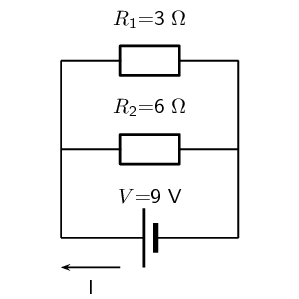There are two resistors in parallel so we calculate the equivalent resistance of the combination:

\begin{align*} \frac{1}{R_{p}} & = \frac{1}{R_{1}} + \frac{1}{R_{2}} \\ & = \frac{1}{3} + \frac{1}{6} \\ & = \frac{2}{6} + \frac{1}{6} \\ & = \frac{3}{6} \\ R_{p} & = \text{2}\text{ Ω} \end{align*}

Now we can use Ohm's law to find the current:

\begin{align*} I & = \frac{V}{R} \\ & = \frac{9}{2} \\ & = \text{4,5}\text{ A} \end{align*}

Two ohmic resistors are connected in series. The resistance of the one resistor is $$\text{4}$$ $$\text{Ω}$$. What is the resistance of the other resistor if a current of $$\text{0,5}$$ $$\text{A}$$ flows through the resistors when they are connected to a voltage supply of $$\text{6}$$ $$\text{V}$$

We first use Ohm's law to find the total resistance:

\begin{align*} R & = \frac{V}{I} \\ & = \frac{\text{6}}{\text{0,5}} \\ & = \text{12}\text{ Ω} \end{align*}

Now we can find the resistance of the other resistor:

\begin{align*} R_{s} & = R_{1} + R_{2} \\ 12 & = 4 + R_{2} \\ R_{2} & = \text{8}\text{ Ω} \end{align*}

Determine the equivalent resistance of the following circuits: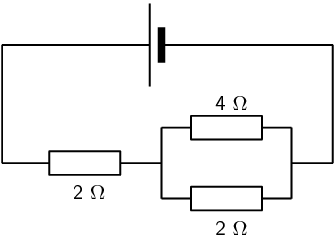We first determine the resistance of the parallel component:

\begin{align*} \frac{1}{R_{p}} & = \frac{1}{R_{1}} + \frac{1}{R_{2}} \\ & = \frac{1}{4} + \frac{1}{2} \\ & = \frac{3}{4} \\ R_{p} &= \frac{4}{3}\text{Ω} \end{align*}

Now we can calculate the total resistance:

\begin{align*} R_{s} & = R_{3} + R_{p} \\ & = 2 + \frac{4}{3} \\ & = \frac{10}{3}\text{Ω} \end{align*}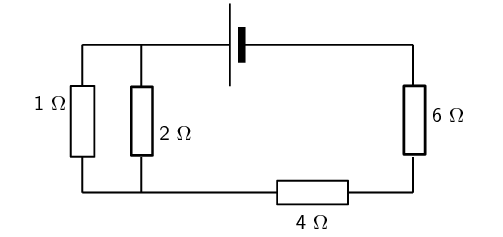We first calculate the equivalent resistance of the parallel part:

\begin{align*} \frac{1}{R_{p}} & = \frac{1}{R_{1}} + \frac{1}{R_{2}} \\ & = \frac{1}{2} + \frac{1}{1} \\ & = \frac{3}{2} \\ R_{p} &= \frac{2}{3}\text{Ω} \end{align*}

Now we calculate the total resistance:

\begin{align*} R_{s} & = R_{p} + R_{3} + R_{4} \\ & = \frac{2}{3} + 4 + 6 \\ & = \text{10,67}\text{ Ω} \end{align*}

The diagram shows an electric circuit consisting of a battery and four resistors.

The potential difference (voltage) over the battery is $$V_A = \text{1,2}\text{ V}$$

The the resistors are rated as follows:

• $$R_1 = \text{4,2}\text{ Ω}$$
• $$R_2 = \text{2,9}\text{ Ω}$$
• $$R_3 = \text{3,8}\text{ Ω}$$
• $$R_4 = \text{3,5}\text{ Ω}$$

Assume that positive charge is flowing in the circuit (conventional current).

Using the concepts of Ohm's law, and electric circuits, determine the following:

What type of circuit is shown in the diagram?

We need to determine whether the circuit is a series, parallel, or combination type of circuit. We do this by looking at how current flows through a circuit.

Recall that current is the movement of electric charge from a higher potential to a lower potential.

We assume that the flowing charge is positive (conventional current). This means that the charges start at the positive terminal of our power source (battery). Here the charges have lots of electrical potential energy.

The charges then move towards the negative terminal through the path that is created by the components and wires of the circuit. During this process, the electrical potential energy is converted into thermal energy by the resistors. Therefore, the charges have less electrical potential energy when they reach the negative terminal of the power source (battery).

The flow of current is indicated by the arrows in the diagram below:

From the diagram it is clear that there is only one path for the current to flow, since the circuit does not split into two or more paths. This means that the current flows through all the components, one after the other (in series). We will label this current $$I_A$$.

The circuit shown in the diagram is a series circuit.

$$0$$

What is the total equivalent resistance $$R_{eq}$$ of the circuit?

• use the values for any physical constants you might need, as listed here

We know that the circuit in the diagram is a series type circuit.

Recall that in a series circuit the equivalent resistance can be calculated by summing the resistance values of the individual resistors:

$R_{eq,S} = R_1 + R_2 + R_3 + \ldots ~~~~~\text{(1)}$

We can rewrite $$\text{(1)}$$ for the circuit in question as:

$R_{eq} = R_1 + R_2 + R_3 + R_4 ~~~~~\text{(2)}$

We can represent this by drawing an equivalent circuit:

We are given the following information:

• the resistance of $$R_1 = \text{4,2}\text{ Ω}$$
• the resistance of $$R_2 = \text{2,9}\text{ Ω}$$
• the resistance of $$R_3 = \text{3,8}\text{ Ω}$$
• the resistance of $$R_4 = \text{3,5}\text{ Ω}$$

Substituting the above values in $$\text{(2)}$$, we get:

\begin{align*} R_{eq} & = R_1 + R_2 + R_3 + R_4\\ & = \text{4,2} + \text{2,9} + \text{3,8} + \text{3,5}\\ & = \text{14,4}\text{ Ω} \end{align*}

Therefore, the total equivalent resistance in the circuit, or $$R_{eq} = \text{14,4}\text{ Ω}$$

$$\text{14,4}$$

What is the potential difference (voltage) across $$R_1$$, or $$V_1$$ ?

• use the values for any physical constants you might need, as listed here

Potential difference, or voltage is a way to describe the difference in electrical potential energy across a component in a circuit. Remember that a resistor converts electrical potential energy into thermal energy, so the electrical potential is higher on the "in" side compared to the "out" side of a resistor.

Ohm's law describes the relationship between the total current $$~I~$$ through an ohmic conductor, its resistance $$R$$, and the potential difference $$V~$$ across it:

$I = \frac{V}{R}~~~~~\text{(3)}$

For the equivalent circuit, we can rewrite $$\text{(3)}$$ as:

$I_A = \frac{V_A}{R_{eq}}~~~~~\text{(4)}$

We are asked to calculate the potential difference $$V_{1}$$ over $$R_{1}$$, as shown in the circuit diagram above, so we use Ohm's law and rewrite $$\text{(3)}$$ to get:

$V_1 = I_1R_1~~~~~\text{(5)}$

Since the circuit in question is a series circuit, we know that the current $$I_{1}$$ flowing through $$R_{1}$$, is the same as the current $$I_{A}$$. This is represented as:

$I_1 = I_A~~~~~\text{(6)}$

Substituting $$\text{(6)}$$ into $$\text{(5)}$$ we get:

$V_1 = I_AR_1~~~~~\text{(7)}$

Substituting $$\text{(4)}$$ into $$\text{(7)}$$ we get:

$V_{1} = I_AR_1 = \left(\frac{V_A}{R_{eq}}\right)R_1 = \frac{V_AR_1}{R_{eq}}~~~~~\text{(8)}$

Recall from Question 2:

$R_{eq} = R_1 + R_2 + R_3 + R_4 ~~~~~\text{(2)}$

Substituting $$\text{(2)}$$ into $$\text{(8)}$$ we get:

$V_{1} = \frac{V_AR_1}{R_1 + R_2 + R_3 + R_4}~~~~~\text{(9)}$

We are given the following information:

• the resistance of $$R_1 = \text{4,2}\text{ Ω}$$
• the resistance of $$R_2 = \text{2,9}\text{ Ω}$$
• the resistance of $$R_3 = \text{3,8}\text{ Ω}$$
• the resistance of $$R_4 = \text{3,5}\text{ Ω}$$
• the potential difference (voltage) over the battery $$V_A = \text{1,2}\text{ V}$$

Substituting the above values in $$\text{(9)}$$, we get:

\begin{align*} V_{1} & = \frac{V_AR_1}{R_1 + R_2 + R_3 + R_4}\\ & = \frac {(\text{1,2})(\text{4,2})} {\text{4,2} + \text{2,9} + \text{3,8} + \text{3,5}}\\ & = 0,35000 \ldots~~~~~\text{(calculated)}\\ & \approx \text{0,350}\text{ V}~~~~~\text{(rounded)} \end{align*}

Therefore, the potential difference (voltage) across $$R_1$$, or $$V_1 \approx \text{0,350}\text{ V}$$.

$$\text{0,350}$$

What is the potential difference (voltage) across $$R_2$$, $$R_3$$, and $$R_4$$, or $$V_2$$, $$V_3$$, and $$V_4$$?

• use the values for any physical constants you might need, as listed here

We are asked to calculate the three remaining potential differences, $$V_2$$, $$V_3$$, and $$V_4$$. This is shown in the diagram below:

We will use the same method to obtain three expression for $$V_2$$, $$V_3$$, and $$V_4$$ as was used in Question 3 for $$V_1$$. We get:

$V_{2} = \frac{V_AR_2}{R_1 + R_2 + R_3 + R_4}~~~~~\text{(10)}$

$V_{3} = \frac{V_AR_3}{R_1 + R_2 + R_3 + R_4}~~~~~\text{(11)}$

$V_{4} = \frac{V_AR_4}{R_1 + R_2 + R_3 + R_4}~~~~~\text{(12)}$

We are given the following information:

• the resistance of $$R_1 = \text{4,2}\text{ Ω}$$
• the resistance of $$R_2 = \text{2,9}\text{ Ω}$$
• the resistance of $$R_3 = \text{3,8}\text{ Ω}$$
• the resistance of $$R_4 = \text{3,5}\text{ Ω}$$
• the potential difference (voltage) over the battery $$V_A = \text{1,2}\text{ V}$$

Substituting the above values in $$\text{(10)}$$, $$\text{(11)}$$, and $$\text{(12)}$$ we get:

\begin{align*} V_{2} & = \frac{V_AR_2}{R_1 + R_2 + R_3 + R_4}\\ & = \frac {(\text{1,2})(\text{2,9})} {\text{4,2} + \text{2,9} + \text{3,8} + \text{3,5}}\\ & = 0,24167 \ldots~~~~~\text{(calculated)}\\ & \approx \text{0,242}\text{ V}~~~~~\text{(rounded)} \end{align*}

\begin{align*} V_{3} & = \frac{V_AR_3}{R_1 + R_2 + R_3 + R_4}\\ & = \frac {(\text{1,2})(\text{3,8})} {\text{4,2} + \text{2,9} + \text{3,8} + \text{3,5}}\\ & = 0,31667 \ldots~~~~~\text{(calculated)}\\ & \approx \text{0,317}\text{ V}~~~~~\text{(rounded)} \end{align*}

\begin{align*} V_{4} & = \frac{V_AR_4}{R_1 + R_2 + R_3 + R_4}\\ & = \frac {(\text{1,2})(\text{3,5})} {\text{4,2} + \text{2,9} + \text{3,8} + \text{3,5}}\\ & = 0,29167 \ldots~~~~~\text{(calculated)}\\ & \approx \text{0,292}\text{ V}~~~~~\text{(rounded)} \end{align*}

Therefore, the potential difference (voltage) across $$R_2$$, or $$V_2 \approx \text{0,242}\text{ V}$$.

The potential difference (voltage) across $$R_3$$, or $$V_3 \approx \text{0,317}\text{ V}$$.

The potential difference (voltage) across $$R_4$$, or $$V_4 \approx \text{0,292}\text{ V}$$.

$$\text{0,242}$$ $$\text{0,317}$$ $$\text{0,292}$$